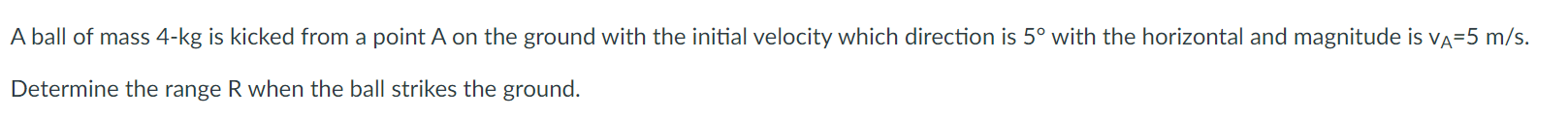Home / Expert Answers / Mechanical Engineering / dynamics-a-ball-of-mass-4-mathrm-kg-is-kicked-from-a-point-a-on-the-ground-with-the-i-pa355

# (Solved): Dynamics A ball of mass $$4-\mathrm{kg}$$ is kicked from a point $$A$$ on the ground with the i ...Dynamics

A ball of mass $$4-\mathrm{kg}$$ is kicked from a point $$A$$ on the ground with the initial velocity which direction is $$5^{\circ}$$ with the horizontal and magnitude is $$\mathrm{v}_{\mathrm{A}}=5 \mathrm{~m} / \mathrm{s}$$. Determine the range $$\mathrm{R}$$ when the ball strikes the ground.

We have an Answer from Expert